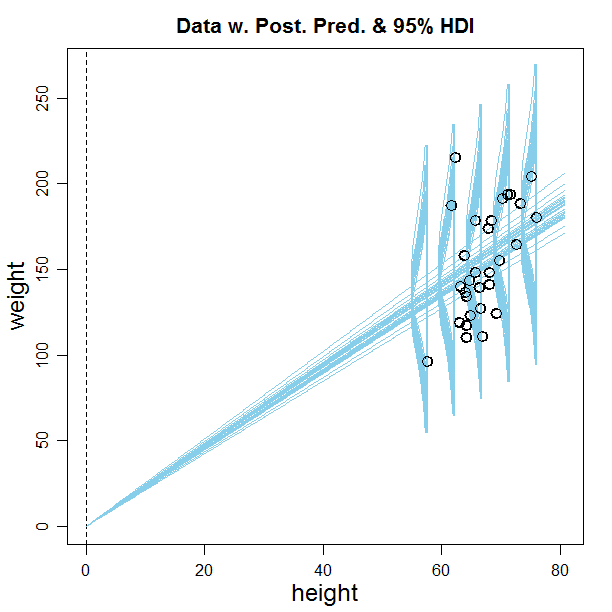## Monday, June 27, 2016

### Brexit: "Bayesian" statistics renamed "Laplacian" statistics

With the U.K. leaving the E.U., it's time for "Bayesian" to exit its titular role and be replaced by "Laplacian".

;-)

Various historians (e.g., Dale, 1999; McGrayne, 2011; as cited in DBDA2E) have argued that despite Bayes and Price having published the basic formula first, it was Laplace who did all the heavy lifting to develop the methods. Laplace was French, while Bayes and Price were English.

On the other hand, Bayes did attend the University of Edinburgh, and lived around London, so maybe he would have voted to stay!

This humorous implication of Brexit was suggested by a participant at a workshop I presented in St. Gallen, Switzerland, last week, after I briefly described the history above and showed the book photographed at Bayes' tomb and at Laplace's memorial. I have forgotten who it was that made the joke. If the originator of the joke is reading this, please put a comment below or send me an email!

## Thursday, June 23, 2016

### Fixing the intercept at zero in Bayesian linear regression

In DBDA2E and in workshops, I present an example of simple linear regression: predicting an adult's weight from his/her height. A participant at a recent workshop suggested that maybe the y-intercept should be fixed at zero, because a person of zero height should have zero weight. I replied that the linear trend is really only intended as a description over the range of reasonable adult heights, not to be extrapolated all the way to a height of zero. Nevertheless, in principle it would be easy to make the restriction in the JAGS model specification. But then it was pointed out to me that the JAGS model specification in DBDA2E standardizes the variables -- to make the MCMC more efficient -- and setting the y intercept of the standardized y to zero is (of course) not the same as setting the y intercept of the raw scale to zero. This blog post shows how to set the y intercept on the raw scale to zero.

For reference, here (below) is the original model specification that does not fix the y intercept at zero. (This is in file Jags-Ymet-Xmet-Mrobust.R.) Notice two lines below in bold font and yellow highlight, regarding the prior on the standardized intercept zbeta0 and the transformation back to the raw-scale intercept beta0:

# Standardize the data:
data {
Ntotal <- length(y)
xm <- mean(x)
ym <- mean(y)
xsd <- sd(x)
ysd <- sd(y)
for ( i in 1:length(y) ) {
zx[i] <- ( x[i] - xm ) / xsd
zy[i] <- ( y[i] - ym ) / ysd
}
}
# Specify the model for standardized data:
model {
for ( i in 1:Ntotal ) {
zy[i] ~ dt( zbeta0 + zbeta1 * zx[i] , 1/zsigma^2 , nu )
}
# Priors vague on standardized scale:
zbeta0 ~ dnorm( 0 , 1/(10)^2 )
zbeta1 ~ dnorm( 0 , 1/(10)^2 )
zsigma ~ dunif( 1.0E-3 , 1.0E+3 )
nu ~ dexp(1/30.0)
# Transform to original scale:
beta1 <- zbeta1 * ysd / xsd
beta0 <- zbeta0 * ysd  + ym - zbeta1 * xm * ysd / xsd
sigma <- zsigma * ysd
}

Now how to fix beta0 to zero: Instead of putting a broad prior distribution on zbeta0, it is set to whatever value would make raw-scale beta0 equal zero. To determine that corresponding value of zbeta0, we use the transform-to-raw-scale formula for beta0, shown at the end of the model specification above. In that formula, set beta0 to 0, then solve for zbeta0. Replace the prior on zbeta0 as follows:

# zbeta0 ~ dnorm( 0 , 1/(10)^2 )
# Fix y intercept at zero:
zbeta0 <- zbeta1*xm/xsd - ym/ysd

When run on the height/weight data (using script Jags-Ymet-Xmet-Mrobust-Example.R), a smattering of regression lines from the posterior distribution looks like this:You can see that the y-intercept has indeed been fixed at zero for all the regression lines.

One crucial trick to getting the graphs to display: In the plotMCMC command of Jags-Ymet-Xmet-Mrobust-Example.R, set showCurve to TRUE:

plotMCMC( mcmcCoda , data=myData , xName=xName , yName=yName ,
compValBeta1=0.0 , ropeBeta1=c(-0.5,0.5) ,
pairsPlot=TRUE , showCurve=TRUE ,
saveName=fileNameRoot , saveType=graphFileType )

If showCurve=FALSE, then it tries to make a histogram which fails for the weird values of beta0 generated by the MCMC.